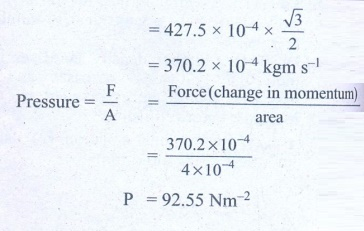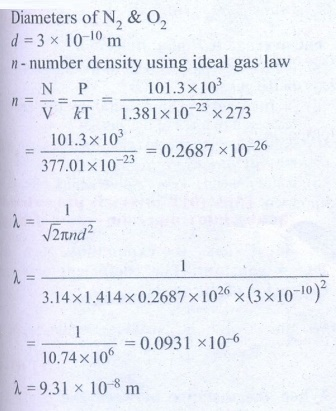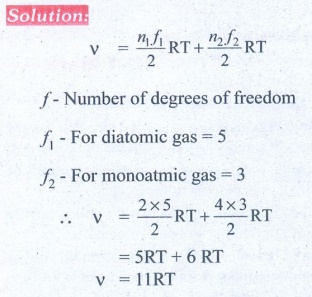Home | Kinetic Theory of Gases: Important Questions

# Kinetic Theory of Gases: Important Questions

1. What is the microscopic origin of pressure?

2. What is the microscopic origin of temperature?

3. Why moon has no atmosphere?

4. Write the expression for rms speed, average speed and most probable speed of a gas molecule.

5. What is the relation between the average kinetic energy and pressure?

6. Define the term degrees of freedom.

7. State the law of equipartition of energy.

8. Define mean free path and write down its expression.

9. Deduce Charles’ law based on kinetic theory.

10. Deduce Boyle’s law based on kinetic theory.

11. Deduce Avogadro’s law based on kinetic theory.

12. List the factors affecting the mean free path.

13. What is the reason for Brownian motion?

1. Write down the postulates of kinetic theory of gases.

2. Derive the expression of pressure exerted by the gas on the walls of the container.

3. Explain in detail the kinetic interpretation of temperature.

4. Describe the total degrees of freedom for monoatomic molecule, diatomic molecule and triatomic molecule.

5. Derive the ratio of two specific heat capacities of monoatomic, diatomic and triatomic molecules

6. Explain in detail the Maxwell Boltzmann distribution function.

7. Derive the expression for mean free path of the gas.

8. Describe the Brownian motion.

### Numerical Problems

1. A fresh air is composed of nitrogen N2(78%) and oxygen O2(21%). Find the rms speed of N2 and O2 at 20°C.

Solution:

For Nitrogen,

Molar mass m = 0.0280 kg/mol

Temperature T = 20°C = 20 + 273 = 293KAns: For vrms = 511 m s-1

For O2vrms = 478 m s-1

2. If the rms speed of methane gas in the Jupiter’s atmosphere is 471.8 m s-1, show that the surface temperature of Jupiter is sub-zero.

Solution:

RMS speed of methane gas = 471.8 ms-1 =Vrms

Sub-zero temperature Molar mass of methane gas

= 16.04 × 10-3 kg mol-1

Surface temperature of Jupiter T = ?

Gas constant R =8.31Ans: -130°C

3. Calculate the temperature at which the rms velocity of a gas triples its value at S.T.P.Ans: T1 = 273 K, T2 = 2457 K

4. A gas is at temperature 80°C and pressure 5 × 10-10N m-2. What is the number of molecules per m3 if Boltzmann’s constant is 1.38 × 10-23 J K-1

Solution:

Temperature of gas T = 80°C = 80 + 273 = 353K

Pressure of gas P = 5 × 10-10 Nm-2

Boltzmann’s constant k = 1.38 × 10-23 Jk-1

Volume of gas V = 1 m3

No. of molecules n = ?Ans: 1.02 × 1011

5. From kinetic theory of gases, show that Moon cannot have an atmosphere (Assume k = 1.38 × 10-23 J K-1 Temperature T=0°C=273K).

Ans: vescape = vrms= 1.86 km s-1

6. If 1020 oxygen molecules per second strike 4 cm2 of wall at an angle of 30° with the normal when moving at a speed of 2 × 103 m s-1, find the pressure exerted on the wall. (mass of 1 atom = 1.67 × 10-27 kg)

Solution

Mass of 1 O2 atom = 2.67 × 10-26 kg

Mass of 1020 O2 atom = 2.67 × 10-26 kg

= 26.72 × 10-27 × 1020

Momentum P = mv

= 26.72 × 10-7 × 8 × 2 × 103

= 427.5 × 10-4 kgm s-1

Component of momentum normal to wall is 30°

= 427.5 ×10-4 × cos 30° .Ans: 92.4 N m-2

7. During an adiabatic process, the pressure of a mixture of monatomic and diatomic gases is found to be proportional to the cube of the temperature. Find the value of γ = (Cp/CV)

SolutionAns: 3/2

8. Calculate the mean free path of air molecules at STP. The diameter of N2 and O2 is about 3 × 10-10 m

SolutionAns: λ≈9 × 10-8 m

9. A gas made of a mixture of 2 moles of oxygen and 4 moles of argon at temperature T. Calculate the energy of the gas in terms of RT. Neglect the vibrational modes.Ans: 11RT

10. Estimate the total number of air molecules in a room of capacity 25 m3 at a temperature of 27°C.

Solution:

Volume of the room V = 25.0m3

Temperature of the room T = 27°C = 300K

Pressure in the room P = estimate

= 1 × 1.013 × 105 Pa

The ideal gas equation relating pressure (P), volume (v) absolute temperature (T) can be written is

PV = KB NTAns: 6.1 × 1026 molecules

Tags : Physics , 11th Physics : UNIT 9 : Kinetic Theory of Gases
Study Material, Lecturing Notes, Assignment, Reference, Wiki description explanation, brief detail
11th Physics : UNIT 9 : Kinetic Theory of Gases : Kinetic Theory of Gases: Important Questions | Physics